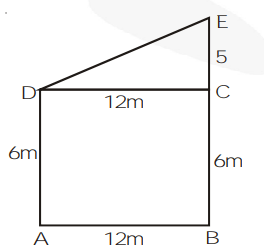# Two poles of height 6 m and 11 m stand on a plane ground.Question.

Two poles of height 6 m and 11 m stand on a plane ground. If the distance between the feet of the poles is 12 m, find the distance between their tops.

Solution:Let AD and BE be two poles of height 6 m and 11 m and AB = 12 m

In $\triangle \mathrm{DEC}$, by pythagoras theorem

$\mathrm{DE}^{2}=\mathrm{CD}^{2}+\mathrm{CE}^{2}$

$\mathrm{DE}^{2}=12^{2}+5^{2}(\mathrm{DC}=\mathrm{AB}=12 \mathrm{~m})$

$\mathrm{DE}=\sqrt{144+25}=\sqrt{169}=13 \mathrm{~m}$

Thus, distance between their tops is 13 m